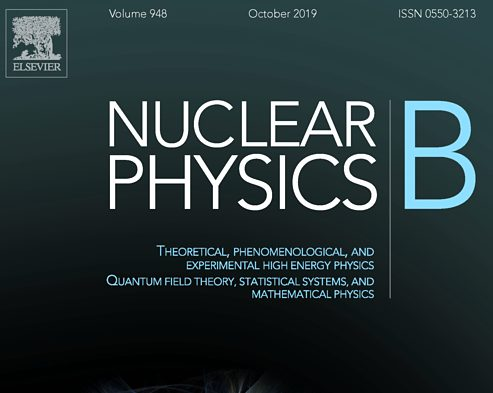In this work an algorithm to derive field theoretical models of polymers under topological constraints is presented. For a wide class of topological invariants, this new strategy allows to obtain, just following a set of easy-to-implement rules, a field theory describing the statistical mechanics of linked polymer rings. The interactions between the monomers arising due to the topological constraints are mediated by suitable Abelian vector fields. This algorithm provides a great advantage with respect to the previous approaches, in which the implementation of a given topological invariant T in polymer models requires the construction of an ad-hoc topological field theory. This is not an easy task for topological invariants that are more refined than the Gauss linking number. In the already known case of the Gauss linking number, the equivalence between the new approach and the former ones is shown.
Other results of this work are:
– Extension of the algorithm to take into account of the vacuum expectation values (vevs) of any observable. Before, it was not easy to translate in the language of field theories observables like the gyration radius. Only the partition function of the system and the so-called moments of the topological invariant T could be considered;
– Reduction of the calculation of the vevs of the moments of T to the evaluation of a free field theory amplitude
– Sketch of a perturbation scheme to compute the vevs of microscopic models of linked polymer rings